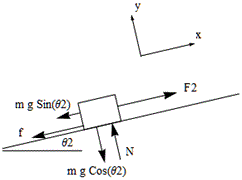In: Physics

# A cart for hauling ore out of a gold mine has a mass of 423 kg,...

A cart for hauling ore out of a gold mine has a mass of 423 kg, including its load. The cart runs along a straight stretch of track that is sloped 4.51

## Solutions

##### Expert Solution

givent aht

mass m = 423 kg
coeffiecient of friction ? = 0.0127
accleration due to gravity g = 9.8 m/s2
inclied angel ?2 = 4.51
inclined angel ?1 = 13.1
distance travelled d = 159 m
applied fore F = 535 N

the component of the force from the donkey in the direction of motion is

F2 = F1 cos?1
=521.08 N
from the free body diagram?Fy = N - mg cos?2 = 0
N = mg cos?2
= 4132.56 N
?Fx = F2 - mg sin?2 - f = ma
here   f = ? N
= 52.48
F2 - mg sin?2 - f = ma
a = F2 - mg sin?2 - f/ m
= 0.337 m/s^2
work done by donkey
W = F2 d
=82851.72 J
work done by gravity
W = - mg sin?2 d
= -51828.53 J
work done by friction W = - fd
= - 8344.32 J

## Related Solutions

##### A frictionless cart of mass ? = 4.00 kg has a shape of a ramp with...
A frictionless cart of mass ? = 4.00 kg has a shape of a ramp with an incline angle ? = 60.0°. The ramp is pulled by a constant horizontal force ? = 15.0 N. Another frictionless cart of mass? = 2.00 kg can roll along the ramp and is attached to the top of the ramp at point ? by a spring of constant ? = 300. N/m. The spring is free to pivot without friction around point ?....
##### 4) Two carts collide on a level track. Cart A has mass of 3 kg and...
4) Two carts collide on a level track. Cart A has mass of 3 kg and cart B has mass of 5 kg. Before the collision cart A moves towards stationary cart B with the speed of 5 m/s. a) What is the momentum of the system of two carts before the collision? b) What is the kinetic energy of the system of two carts before the collision? c) What is the momentum of the system of two carts after...
##### EXCEL SOLVER: A gold processor has two sources of gold ore, source A and source B....
EXCEL SOLVER: A gold processor has two sources of gold ore, source A and source B. In order to keep his plant running, at least three tons of ore must be processed each day. Ore from source A costs $20 per ton to process, and ore from source B costs$10 per ton to process. Costs must be kept to less than \$80 per day. Moreover, Federal Regulations require that the amount of ore from source B cannot exceed twice...